##### Linear Algebra For Dummies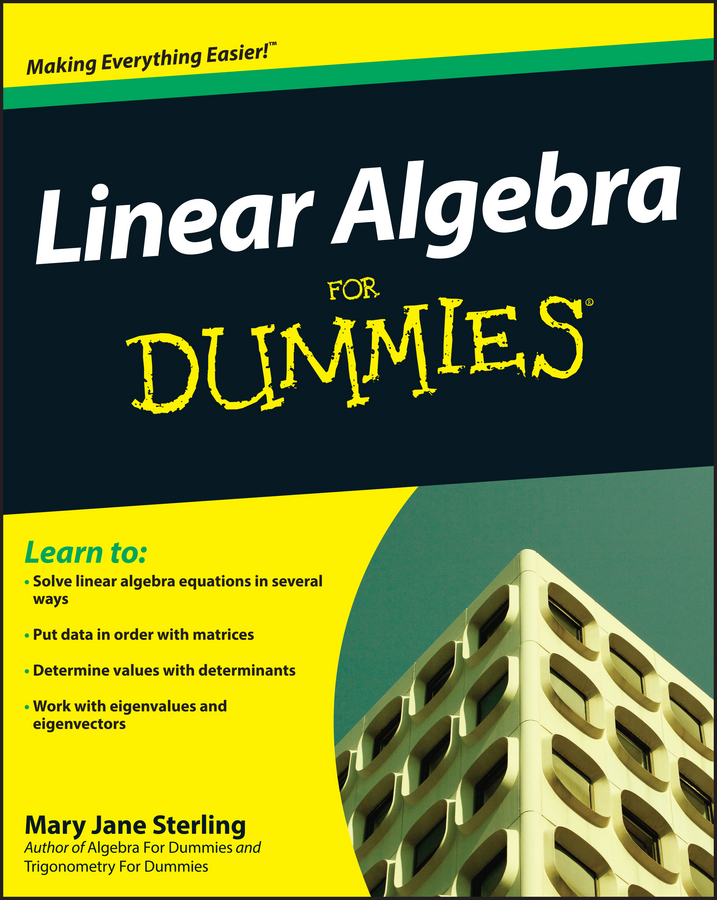When you begin combining functions (like adding a polynomial and a square root, for example), the domain of the new combined function is affected. The same can be said for the range of a combined function; the new function will be based on the restriction(s) of the original functions.

The domain is affected when you combine functions with division because variables end up in the denominator of the fraction. When this happens, you need to specify the values in the domain for which the quotient of the new function is undefined. The undefined values are also called the excluded values for the domain. If f(x) = x2 – 6x + 1 and g(x) = 3x2 – 10, if you look at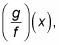this fraction has excluded values because f(x) is a quadratic equation with real roots. The roots of f(x) are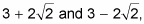so these values are excluded.

Unfortunately, there isn’t one foolproof method for finding the domain and range of a combined function. The domain and range you find for a combined function depend on the domain and range of each of the original functions individually. To get an idea of the domain and range of the combined function, you simply break down the problem and look at the individual domains and ranges.

## Finding the domain of a composition of functions

Given two functions, f(x) and g(x), assume you have to find the domain of the new combined function f(g(x)). To do so, you need to find the domain of each individual function first. If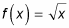and g(x) = 25 – x2, here’s how you find the domain of the composed function f(g(x)):

1. Find the domain of f(x).

Because you can’t square root a negative number, the domain of f has to be all non-negative numbers. Mathematically, you write this as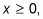or in interval notation,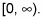2. Find the domain of g(x).

Because this equation is a polynomial, its domain is all real numbers, or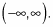3. Find the domain of the combined function.

When specifically asked to look at the composed function f(g(x)), note that g is inside f. You’re still dealing with a square root function, meaning that all the rules for square root functions still apply. So the new radicand of the composed function has to be non-negative: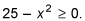Solving this quadratic inequality gives you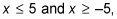which make up the domain of the composed function: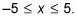## Finding the range of a composition of functions

To find the range of the same composed function, you must also consider the range of both original functions first:

1. Find the range of f(x).

A square-root function always gives non-negative answers, so its range is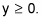2. Find the range of g(x).

This function is a polynomial of even degree (specifically, a quadratic), and even-degree polynomials always have a minimum or a maximum value. The higher the degree on the polynomial, the harder it is to find the minimum or the maximum. Because this function is “just” a quadratic, you can find its min or max by locating the vertex.

First, rewrite the function as g(x) = –x2 + 25. This form tells you that the function is a transformed quadratic that has been shifted up 25 and turned upside down. Therefore, the function never gets higher than 25 in the y direction. The range is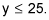3. Find the range of the composed function f(g(x)).

The function g(x) reaches its maximum (25) when x = 0. Therefore, the composed function also reaches its maximum at x = 0: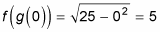The range of the composed function has to be less than that value, or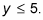Remember that the graph of this combined function also depends on the range of each individual function. Because the range of g(x) must be non-negative, so must be the range of the composed function. This is written as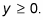Therefore, the range of the composed function is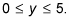If you graph this composed function on your graphing calculator, you get the top half circle of radius 5 that’s centered at the origin.